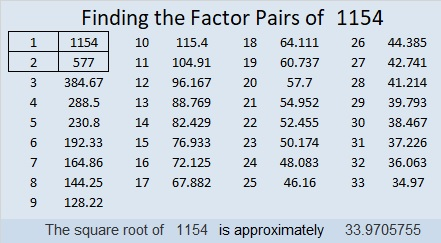# 1154 and Level 5

I’m sure you can have a lot of fun solving this puzzle. Remember to use logic before you write down any of the factors, and it will be fun instead of frustrating.Print the puzzles or type the solution in this excel file: 10-factors-1148-1160

Now I’ll share some facts about the number 1154:

• 1154 is a composite number.
• Prime factorization: 1154 = 2 × 577
• The exponents in the prime factorization are 1 and 1. Adding one to each and multiplying we get (1 + 1)(1 + 1) = 2 × 2 = 4. Therefore 1154 has exactly 4 factors.
• Factors of 1154: 1, 2, 577, 1154
• Factor pairs: 1154 = 1 × 1154 or 2 × 577
• 1154 has no square factors that allow its square root to be simplified. √1154 ≈ 33.970581154 is the sum of eight consecutive prime numbers:
127 + 131 + 137 + 139 + 149 + 151 + 157 + 163 = 1154

25² + 23² = 1154

1154 is the hypotenuse of a Pythagorean triple:
96-1150-1154 calculated from 25² – 23², 2(25)(23), 25² + 23²

1154 is palindrome 202 in BASE 24
because 2(24²) + 2(1) = 2(24² + 1) = 2(577) = 1154

Did you notice that 1154 has a relationship with 23², 24², and 25²?